Impact Factor 2.638 | CiteScore 2.3
More on impact ›

# Frontiers in Physics## Original Research ARTICLE

Front. Phys., 06 November 2020 | https://doi.org/10.3389/fphy.2020.00265

# Floating-Point Calculations on a Quantum Annealer: Division and Matrix Inversion

• 1SavantX, Santa Fe, NM, United States
• 2School of Mathematics, University of Leeds, Leeds, United Kingdom

Systems of linear equations are employed almost universally across a wide range of disciplines, from physics and engineering to biology, chemistry, and statistics. Traditional solution methods such as Gaussian elimination are very time consuming for large matrices, and more efficient computational methods are desired. In the twilight of Moore's Law, quantum computing is perhaps the most direct path out of the darkness. There are two complementary paradigms for quantum computing, namely, circuit-based systems and quantum annealers. In this paper, we express floating point operations, such as division and matrix inversion, in terms of a quadratic unconstrained binary optimization (QUBO) problem, a formulation that is ideal for a quantum annealer. We first address floating point division, and then move on to matrix inversion. We provide a general algorithm for any number of dimensions, and, as a proof-of-principle, we demonstrates results from the D-Wave quantum annealer for 2 × 2 and 3 × 3 general matrices. In principle, our algorithm scales to very large numbers of linear equations; however, in practice the number is limited by the connectivity and dynamic range of the machine.

## 1. Introduction

Systems of linear equations are employed almost universally across a wide range of disciplines, from physics and engineering to biology, chemistry, and statistics. An interesting physics application is computational fluid dynamics (CFD), which requires inverting very large matrices to advance the state of the hydrodynamic system from one time step to the next. An application of importance in biology and chemistry would include the protein folding problem. For large matrices, Gaussian elimination and other standard techniques becomes too time consuming, and faster computational methods are therefore desired. As Moore's Law draws to a close, quantum computing offers the most direct path forward; it is also perhaps the most radical path. In a nutshell, quantum computers are physical systems that exploit the laws of quantum mechanics to perform arithmetic and logical operations much faster than a conventional computer. In the words of Harrow, Hassidim, and Lloyd (HHL) , “quantum computers are devices that harness quantum mechanics to perform computations in ways that classical computers cannot.” There are currently two complementary paradigms for quantum computing, namely, circuit-based systems and quantum annealers. Circuit-based systems exploit the deeper properties of quantum mechanics such coherence, entanglement, and non-locality, while quantum annealers mainly take advantage of tunneling between metastable states and the ground state. In , HHL introduces a circuit-based method by which the inverse of a matrix can be computed, and [2, 3] provide implementations of the algorithm to invert 2 × 2 matrices. Circuit-based methods are limited by the relatively small number of qubits that can be entangled into a fully coherent quantum state, currently of order 50 or so. An alternative approach to quantum computing is the quantum annealer , which takes advantage of quantum tunneling between metastable states and the ground state. The D-Wave Quantum Annealers have reached capacities of 2000+ qubits, which suggests that quantum annealers could be quite effective for linear algebra with hundreds to thousands of degrees of freedom. In this paper, we express floating point operations such as division and matrix inversion as quadratic unconstrained binary optimization (QUBO) problems, which are ideal for a quantum annealer. We should mention that our algorithm provides the full solution the matrix problem, while HHL provides only an expectation value. Furthermore, our algorithm places no constraints on the matrix that we are inverting, such as a sparsity condition.

The first step in mapping a general problem to a QUBO problem begins with constructing a Hamiltonian that encodes the problem in terms of a set of “logical” qubits. Next, because of the limited connectivity of the D-Wave chip, it will be necessary to “embed” the problem onto the chip, first by mapping each logical qubit to a collection or “chain” of physical qubits and then by determining parameter settings for all the physical qubits, including the chain couplings. We have implemented our algorithms on the D-Wave 2000Q and 2X chips, illustrating that division and matrix inversion can indeed be performed on an existing quantum annealer. The algorithms that we propose should ideally scale well for large numbers of equations, and should be applicable to a matrix inversion of relatively high order (although probably not exponentially higher order as in HHL). Currently, the scaling that may be achieved is limited by the connectivity and dynamic range of the chip.

Before examining the various algorithms, it is useful to review the basic formalism and to establish some notation. The general problem starts with a graph ${G}$ = (${V}$, ${E}$), where ${V}$ is the vertex set and ${E}$ is the edge set. The QUBO Hamiltonian on ${G}$ is defined by

with Qr ∈ {0, 1} for all r${V}$. The coefficient Ar is called the weight at vertex r, while the coefficient Brs is called the strength between vertices r and s. It might be better to call (1.1) the objective function rather than the Hamiltonian, as H${G}$ is a real-valued function and not an operator on a Hilbert-space. However, it is easy to map (1.1) in an equivalent Hilbert space form,

where ${\stackrel{^}{Q}}_{r}|Q〉={Q}_{r}|Q〉$ for all r${V}$, and |Q〉 ∈ ${H}$ for Hilbert space ${H}$. The hat denotes an operator on the Hilbert space, and Qr is the corresponding Eigenvalue of ${\stackrel{^}{Q}}_{r}$ with Eigenstate |Q〉. Consequently, we can write

and we use the terms Hamiltonian and objective function interchangeably. By the QUBO problem, we mean the problem of finding the lowest energy state |Q〉 of the Hamiltonian (1.2), which corresponds to minimizing Equation (1.1) with respect to the Qr. This is an NP-hard problem uniquely suited to a quantum annealer. Rather than sampling all 2#${V}$ possible states, quantum tunneling finds the most likely path to the ground state by minimizing the Euclidian action. In the case of the D-Wave 2X chip, the number of distinct quantum states is of order the very large number 21000, and the ground state is selected from this jungle of quantum states by tunneling to those states with a smaller Euclidean action.

The Ising model  is perhaps the quintessential physical example of a QUBO problem, and, indeed, it is one of the most studied systems in statistical physics. The Ising model consists of a square lattice of spin-1/2 particles with nearest neighbor spin–spin interactions between sites r and s, and when the system is immersed in a nonuniform magnetic field, this introduces coupling terms at individual sites r, thereby producing a Hamiltonian of the form

where Σr = ±1/2. The Ising problem is connected to the QUBO problem by Σr = Qr − 1/2.

For floating point division to R bits of resolution, the graph ${G}$ is in fact just the fully connected graph KR. In terms of vertex and edge sets, we write KR = (${V}$R, ${E}$R), and Figure 1 illustrates K8 and K4. The left panel shows the completely connected graph K8, with vertex and edge sets

while the right panel shows the K4 graph,

FIGURE 1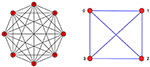Figure 1. The left panel shows the fully connected graph K8, and the right panel shows the corresponding graph K4. To perform a calculation to 8-bit accuracy requires the connectivity of K8. We take the vertex and edge sets of K8 to be ${V}$8 = {0, 1, 2, ⋯, 7} and ${E}$8 = {{0, 1}, {0, 2}, ⋯, {0, 7}, {1, 2}, {1, 3}, ⋯, {6, 7}}. To perform a calculation to 4-bit accuracy requires K4 connectivity, and, similarly, the vertex and edge sets for K4 are ${V}$4 = {0, 1, 2, 3} and ${E}$4 = {{0, 1}, {0, 2}, {0, 3}, {1, 2}, {1, 3}, {2, 3}}.

Just as 8-bit is called a word, 4-bit is called a nibble. As we will also see, the dynamic range of the D-Wave is most directly suitable to K4, and the connectivity of K4 consequently gives a quantum nibble.

Let us remark about our summation conventions. Rather than summing over the edges,

we find it convenient to sum over all values of r and s taking Brs to be symmetric. In this case, the double sum differs by a factor of two relative to summing over the edge set of the graph,

Furthermore, for r = s, there will be a linear contribution from the idempotency condition ${Q}_{r}^{2}={Q}_{r}$, so that

We can write this as

## 2. Floating Point Division on a Quantum Annealer

### 2.1. Division as a QUBO Problem

In this section we present an algorithm for performing floating point division on a quantum annealer. Given two input parameters m and y to R-bits of resolution, the algorithm calculates the ratio y/m to R bits of resolution. The corresponding division problem can be represented by the linear equation

which has the unique solution

Solving (2.1) on a quantum annealer amounts to finding an objective function H(x) whose minimum corresponds to the solution of Equation (2.2). Although the form of H(x) is not unique, for this work we employ the simple real-valued quadratic function

where m and y are continuous parameters. For an ideal annealer, we do not have to concern ourselves with the numerical range and resolution of the parameters m and y; however, for a real machine such as the D-Wave, this is an important consideration. For a well-conditioned matrix, we require that the parameters m and y possess a numerical range that spans about an order of magnitude, from approximately 0.1–1.0. This provides about 3–4 bits of resolution: 1/20 = 1, 1/21 = 0.5, 1/22 = 0.25, and 1/23 = 0.125. The dynamic range and the connectivity both impact the resolution of a calculation.

To proceed, let us formulate floating point division as a quadratic unconstrained binary optimization (QUBO) problem. The algorithm starts by converting the real-valued number x in (2.3) into an R-bit binary format, while the numbers m and y remain real valued parameters of the objective function. For any number χ ∈ [0, 2), the binary representation accurate to R bits of resolution can be expressed by [Q0.Q1Q2QR−1]2, where Qr ∈ {0, 1} is value of the r-th bit, and the square bracket indicates the binary representation1. It is more algebraically useful to express this in terms of the power series in 2r,

In order to represent negative number, we perform the binary offset

where x ∈ [−1, 3). The objective function now takes the form

The constant term (m + y)2 can be dropped when finding the minimum of (2.6), but we choose to keep it for completeness. Equation (2.4) provides a change of variables χ = χ[Q] (where Q is the collection of the Qr), and this allows us to express (2.3) in the form

In the notation of graph theory, we would write

where ${V}$R = {0, 1, 2, ⋯, R − 1} is the vertex set, and ${E}$R is the edge set. We often employ an abuse of notation and write rs${E}$R to mean {r, s} ∈ ${E}$R. Thus, instead of B{r, s}, we write Brs. Since the order of the various elements of a set are immaterial, we require Brs to be symmetric in r and s. Rather than summing over the edge sets rs${E}$R, we employ the double sum $\sum _{r\ne s}$, which introduces a relative factor of two in the convention for the strengths Brs. The goal of this section is to find Ar and Brs in terms of m and y.

Note that we can generalize the simple binary offset (2.4) if we scale and shift χ ∈ [0, 2) by

so that x ∈ [−d, 2cd). When d > 0 and c > d/2, the domain of x always contains a positive and negative region, and the precise values for d and c can be chosen based on the specifics of the problem. For Equation (2.9), the objective function takes the form

For simplicity of notation, this paper employs the simple binary offset (2.5), although our Python interface to the D-Wave quantum annealer employs the generalized form (2.10).

Equation (2.4) allows us to express the quadratic term in χ as

where we have used the idempotency condition ${Q}_{r}^{2}={Q}_{r}$ along the diagonal in the last term of (2.11). Substituting the forms (2.4) and (2.11) into (2.6) yields the Hamiltonian

and the Ising coefficients in (2.7) can be read off:

Because of the double sum over r and s in the objective function in (2.12), the algorithm requires a graph of connectivity KR. The special cases of K8 and K4 have been illustrated in Figure 1. To obtain higher accuracy than the KR graph allows, we can iterate this procedure in the following manner. Suppose we start with y0 = y and are given a value yn−1 with n > 1; we then advance the iteration to yn in the following manner:

Now that we have the value yn, we can repeat the process to find yn+1, and we can stop the iterative procedure when the desired level of accuracy has been achieved.

### 2.2. Embedding KR Onto the D-Wave Chimera Architecture

The D-Wave Chimera chip consists of coupled bilayers of micro rf-SQUIDs overlaid in such a way that, while relatively easy to fabricate, results in a fairly limited set of physical connections between the qubits. However, by chaining together well chosen qubits in a positively correlated manner, this limitation can largely be overcome. The process of chaining requires that we (i) embed the logical graph onto the physical graph of the chip (for example K4 into C8) and that we (ii) assign weights and strengths to the physical graph embedding in such as a way as to preserve the ground state of the logical system. These steps are called graph embedding and Hamiltonian embedding, respectively.

Let us explore the connectivity of the D-Wave Chimera chip in more detail. The D-Wave architecture employs the C8 bipartate Chimera graph as its most basic unit of connectivity. This unit cell is illustrated in Figure 2, and it consists of 8 qubits connected in a 4 × 4 bipartate manner. The left panel of the figure uses a column format in laying out the qubits, and the right panel illustrates the corresponding qubits in a cross format, where the gray lines represent the direct connections between the qubits. The cross format is useful since it minimizes the number intersecting connections. The complete two-dimensional chip is produced by replicating C8 along the vertical and horizontal directions, as illustrated in Figure 3, thereby providing a chip with thousands of qubits. The connections between qubits are limited in two ways: (i) by the connectivity of the basic unit cell C8 and (ii) by the connectivity between the unit cells across the chip. The bipartate graph C8 = (${V}$8, ${B}$8) is formally defined by the vertex set ${V}$8 = {1, 2, ⋯, 8}, and the edge set

FIGURE 2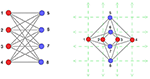Figure 2. The left panel illustrates the bipartate graph C8 in column format, while the right panel illustrates the corresponding graph in cross format, often called a Chimera graph. The gray lines represent direct connections between qubits. The cross format is useful since it minimizes the number intersecting connections. The use of red and blue dots emphasize the bipartate nature of C8, as every red dot is connected to every blue dot, while none of the red and blue dots are connected to one another. The vertex set of C8 is taken to be ${V}$8 = {1, 2, ⋯, 8} and edge set is ${B}$8 = {{1, 5}, {1, 6}, {1, 7}, {1, 8}, {2, 5}, {2, 6}⋯{7, 8}}.

FIGURE 3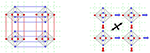Figure 3. The left panel shows the connectivity between four C8 bipartate Chimera zones, and the right panel illustrates how multiple C8 graphs are stitched together along the vertical and horizontal directions to provide thousands of possible qubits. A limitation of this connectivity strategy is that red and blue zones cannot communicate directly with one another, as indicated by the black crossed arrows. The purpose of chaining is to allow communication between the red and blue qubits.

The set ${B}$8 represents the connections between a given red qubit and the corresponding blue qubits in the figures. The red and blue dots illustrate the bipartate nature of C8, as every red dot is connected to every blue dot, while none of the blue and red dots are connected to one another.

We will denote the physical qubits on the D-Wave chip by q. For the D-Wave 2000Q there is a maximum of 2,048 qubits, while the D-Wave 2X has 1,152 qubits. For the example calculation in this text, we only use 10–50 qubits. The physical Hamiltonian or objective function takes the form

where we have introduced a factor of 2 in the strength to account for the symmetric summation over r and s. We will call the qubits Qr of the previous section the logical qubits. To write a program for the D-Wave means finding an embedding of the problem for logical qubits onto the physical collection of qubits q. If the connectivity of the Chimera graphs were large enough, then the logical qubits would coincide exactly with the physical qubits. However, since the graph C8 possesses less connectivity than K4, we must resort to chaining on the D-Wave, even for 4-bit resolution. Figure 4 illustrates the K4 embedding used by our algorithm, where, as before, the left panel illustrates the bipartate graph in column format, and the right panel illustrates the corresponding graph in cross format.

FIGURE 4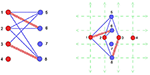Figure 4. The K4 embedding onto C8 used in our implementation of 4-bit of division on the D-Wave. The blue lines represent normal connections between qubits, while the red double-lines represent chained qubits, that is to say, qubits that are strictly correlated (and can thereby represent a single logical qubit at a higher level of abstraction). The qubits 1–6 are chained together, as are the qubits 3–8.

In Figure 4, we have labeled the physical qubits by ℓ = 1, 2, 3⋯8, and we wish to map the problem involving logical qubits Qr Qs Qt onto the four physical qubits q5 q1 q6 q2. The embedding requires that we chain together the two qubits 1-6 and 3-8, respectively. We may omit qubits 4 and 7 entirely. As illustrated in Figure 5, the physical qubits q1 and q6 are chained together to simulate a single logical qubit Qt, while qubits q5 and q2 are mapped directly to the logical qubits Qr and Qs, respectively. Qubit q5 is assigned the weight a5 = Ar and the coupling between q5 and q1 is assigned the value b51 = Brt. Similarly for qubit q2, the vertex is assigned weight a2 = As, and strength between q2 and q6 is b26 = Bst. We must now distribute the logical qubit Qt between q1 and q6 by assigning the values a1, a6 and b16. We distribute the weight At uniformly between qubits q1 and q2, giving a1 = At/2 and a6 = At/2. We must now choose b16. To preserve the energy spectrum, we must shift the values of the weights a1 and a6. We can do this by adding a counter-term Hamiltonian

to the physical Hamiltonian. The double lines in Figures 4, 5 indicate that two qubits are chained together. This means that the qubits are strictly correlated, i.e., when q1 is up then q6 is up, and when q1 is down then q6 is down. This is accomplished by choosing the coupling strength b16 to favor a strict correlation; however, to preserve the ground state energy, this also requires shifting the weights for q1 and q6. For q1 = q6 = 0 we have HCT = 0. We wish to preserve this condition when q1 = q6 = 1, which means 2a + 2b = 0. Furthermore, the state q1 = 1 and q6 = 0 must have positive energy, which means a > 0. Similarly for q1 = 0 and q6 = 1. We therefore choose a1 = a6 = α > 0 and b16 = −α, where α is an arbitrary parameter. This is illustrated in Table 1. A more complicated case is the linear chain of N qubits as shown in Figure 6. The counter-term Hamiltonian is taken to be

FIGURE 5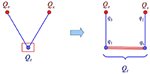Figure 5. The left panel shows three logical qubits Qr, Qs, Qt with connectivity between r-t and t-s. The box surrounding qubit t means that it will be modeled by a linear chain of physical qubits, as illustrated in the right panel. The labeling is taken from Figure 4 for qubits 5-1-6-2, where Qr is mapped to q5, Qs is mapped to q2, and Qt is split between q1 nd q6. Qubits q1 and q6 are chained together to simulate the single logical qubit Qt, while qubits Qr and Qs map directly onto physical qubits q5 and q2.

TABLE 1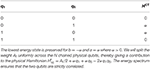Table 1. For two qubits the counter-term Hamiltonian is ${H}^{\text{CT}}\left({q}_{1},{q}_{6}\right)=a{q}_{1}+a{q}_{6}+2b{q}_{1}{q}_{6}$.

FIGURE 6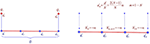Figure 6. Generalization of Figure 5 to a chain of N linear qubits. The right panel illustrates the chain coupling parameters used to create strict correlations of the physical qubits within the chain.

Note that HCT vanishes when qm = 0 for all m = 1⋯N. And conversely, we must arrange the counter-term to vanish when qm = 1. The simplest choice is to take all weights to be the same and all couplings to be identical. Then, to preserve the ground state when the qr = 1, we impose

with α > 0 and r = 1⋯N. The first term in ${a}_{r}^{t}$ distributes the weight At uniformly across all N nodes in the chain. The second set of terms ${b}_{r,r+1}^{t}$ ensures that the qubits of the chain are strictly correlated. The counter-term energy contribution is positive when the linearly chained qubits are not correlated, therefore anti-correlation is always penalized. Table 2 illustrates the spectrum of the counter-term Hamiltonian for three qubits. We may need to choose large values of α, of order 20 or more, to sufficiently separate the states. The uniform spectrum of 4 states with HCT = a in Table 2 arises from a permutation symmetry in q1, q2, q3.

TABLE 2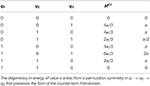Table 2. For a three qubit chain the counter-term Hamiltonian is ${H}^{\text{CT}}\left({q}_{1},{q}_{2},{q}_{3}\right)=a{q}_{1}+a{q}_{2}+a{q}_{3}+2b{q}_{1}{q}_{2}+2b{q}_{2}{q}_{3}$, where a = 4α/3 and b = −α.

To review, note that a linear counter-term is represented in Figure 6. We add a counter-term to break the logical qubits into a chain of physical qubits that preserve the ground state. Let us consider the conditions that we place on the Hamiltonian to ensure strict correlation between the chained qubits. We adjust the values of Ar and Brs to ensure that spin alignment is energetically favorable. By slaving several qubits together, we can overcome the limitations of the Chimera connectivity. As a more complex example, consider the four logical qubits of Figure 7 connected in a circular chain by strengths B12, B24, B43, and B31. Suppose the weights are A1, A2 A3, and A4. Figure 8 provides an example in which each logical qubit is chained in a linear fashion to the physical qubits.

FIGURE 7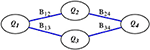Figure 7. Four logical qubits Q1, Q2, Q3, Q4 in a circular loop with connection strengths B12, B24 B43, and B31.

FIGURE 8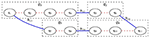Figure 8. A possible mapping of the logical qubits in Figure 7 onto the physical device. Each logical qubit is modeled by a linear chain of strictly correlated qubits.

## 3. Matrix Inversion as a QUBO Problem

In this section we present an algorithm for solving a system of linear equations on a quantum annealer. To precisely define the mathematical problem, let M be a nonsingular N × N real matrix, and let Y be a real N dimensional vector; we then wish to solve the linear equation

The linearity of the system means that there is a unique solution,

and the algorithm is realized by specifying an objective function whose ground state is indeed (3.2). The objective function is not unique, although it must be commensurate with the architecture of the hardware. If the inverse matrix itself is required, it can be constructed by solving (3.1) for each of the N linearly independent basis vectors for Y. It is easy to construct a quadratic objective H(x) whose minimum is (3.2), namely,

In terms of matrix components, this can be written as

Note that ||Y||2 is just a constant, which will not affect the minimization. In principle all constants can be dropped from the objective function, although we choose to keep them for completeness. One may obtain a floating point representation of each component of x = (x1, ⋯, xN)T by expanding in powers of 2 multiplied by Boolean-valued variables ${q}_{r}^{i}\in \left\{0,1\right\}$,

As before, the domains are given by χi ∈ [0, 2) and xi ∈ [−1, 3), and upon expressing x as a function ${q}_{r}^{i}$, we can recast (3.4) in the form

The coefficients are called the weights and the coefficients are the interaction strengths. Note that the algorithm requires a connectivity of KNR for arbitrary matrices.

Let us first calculate the product xixj in (3.4). From (3.5) and (3.6), we find

where we have used the idempotency condition ${\left({q}_{r}^{i}\right)}^{2}={q}_{r}^{i}$ in the second term of (3.9). While the second form is one used by the code, it is more convenient algebraically to use the first form. Substituting (3.8) into the first term in (3.4) gives

The second term in (3.4) can be expressed as

The QUBO coefficients for logical qubits are therefore

In the programming interface, the coefficients are addressed with a 1-dimensional linear index, while the parameters in 3.17 and 3.18 are defined in terms of the 2-dimensional indices i and r, where i = 0, 1, ⋯, N − 1 and r = 0, 1, ⋯, R − 1. Now, we define a 1-1 mapping between these indices and the linear index ℓ = 0, 1, ⋯, N · R − 1. This is just an ordinary linear indexing for 2-dimensional matrix elements, so we choose the usual row-major linear index mapping,

The inverse mapping gives the row and column indices as below,

where ⌊n⌋ is the greatest integer less than or equal to n. The expression “ℓ mod R” is ℓ modulo R. This is a 1-1, invertible mapping between each pair of values of i and r in the matrix index space to every value of ℓ in the linear qubits index space. We can simply replace sums over all index pairs i, r by a single sum over ℓ, provided we also rewrite any isolated indices in i and r as functions of ℓ via their inverse mapping.

We may summarize this observation in the following formal identity. Given some arbitrary quantity, A, that depends functionally upon the tuple (i, r), and possibly upon the individual indices i and r, it is trivial to verify that

where ℓ, i, and r are related as in Equations (3.19)–(3.22). This identity is useful for formal derivations. For example, we may use it to quickly derive the binary expansion of xi in terms of logical qubits. Inserting (3.23) into (3.6) gives,

Clearly, xi has non-zero contributions only for those indices corresponding to i = i = ⌊ℓ/R⌋, that is, only from those qubits within a row in the ${q}_{r}^{i}$ array. Also, those contributions are summed along that row, i.e.,, over r = ℓ mod R. This equation will be used to reconstruct the floating-point solution, x, from the components q of the binary solution returned from the D-Wave annealing runs. The weights and strengths now become

For a 2 × 2 matrix to 4-bit accuracy, we need K8 (4 × 2 = 8), and to 8-bit accuracy we need K16 (8 × 2 = 16). We have inverted matrices up to 3×3 to 4-bit accuracy, which requires K12 (3 × 4 = 12). For an N × N matrix with R bits of resolutions, we must construct linear embeddings of KRN. We could generalize this procedure for complex matrices.

## 4. Calculations

### 4.1. Implementation

The methods above were implemented using D-Wave's Python SAPI interface and tested on a large number of floating-point calculations. Initially, we performed floating-point division on simple test problems with a small resolution. Early on, we discovered that larger graph embeddings tended to produce noisier results. To better understand what was happening we started with a K8 graph embedding to represent two floating-point numbers with only four bits of resolution. Since the D-Wave's dynamic range is limited to about a factor of 10 in the scale of the QUBO parameters, we determined that we could expect no more than 3–4 bits of resolution from any one calculation in any event. However, our binary offset representation (3.5) implies that we should expect no more than 3 bits of resolution in any single run. Indeed, using the K8 embedding, we were able to get exact solutions from the annealer for any division problems that had answers that were multiples of 0.25 between −1.0 and 1.0. Problems in this range that had solutions that were not exact multiples of 0.25 resulted in approximate solutions, effectively “rounded” to the nearest of ±0.25 or ±0.75. At this point we implemented an iterative scheme that uses the current error, or residual, as a new input, keeping track of the accumulated floating-point solution.

The iteration method has been implemented and tested for floating-point division, but we have not yet implemented iteration for matrix inversion. That can be done by using the previous residual (error) as the new inhomogeneous term in the matrix equation. We plan to implement an iterative method in the matrix inversion code eventually. However, we already have good preliminary results on matrix inversion that suggests that this should work reasonably well, at least for well-conditioned matrices. Currently, we are able to solve 2 × 2 and 3 × 3 linear equations involving floating-point numbers up to a resolution of 4 bits, and having well-conditioned matrices, exactly for input vectors with elements defined on [−1, 1] and that are multiples of 0.25. Using an example matrix that is poorly-conditioned, we find that it is generally not possible to get the right answer without first doing some sort of preconditioning to the matrix. But, more importantly, we were able to obtain some insight about why ill-conditioned matrices can be difficult to solve as QUBO problems on a quantum annealer, which gives some hints about how to ameliorate the problem. We will discuss these results, and the effects of ill-conditioning on the QUBO matrix inverse problem below.

#### 4.1.1. Note on Solution Normalization and Iteration

Allowing both the division and linear equation QUBO solvers to work for arbitrary floating-point numbers, and to allow for iterative techniques, requires normalizing the ratio of the current dividend and the divisor, or the residual and matrix, to a value in a range between −1 and 1. For the division problems, we wanted to avoid “dividing in order to divide,” so we normalized each ratio using the difference between the binary exponents of ⌊divisor⌋ and ⌊dividend⌋. These can be found just by using order comparisons, with no explicit divisions. Adding 1 to this yields an “offset”—the largest binary exponent of the ratio—to within a factor of 2 (±1 in the offset), which is sufficient for scaling our QUBO parameters as needed. The fact that our QUBO solutions are always returned in binary representation provides a simple way to bound the solution into a range solvable with the annealer by simply shifting the binary representation of the current dividend by a few bits (using the current offset), which is why we refer to the solution exponent as an “offset.” In this way, the solution can easily be guaranteed to be in the correct range without having to perform any divisions in Python. The “offset” is accumulated and used to construct the current approximation to the floating-point solution on each iteration. The iteration process continues until the error of the approximate solution is less than some tolerance specified by the user.

### 4.2. Results for Division

First, we present some examples for division without iteration. We used a K4 graph embedding for expanding the unknown x up to a resolution of four bits. However, using the binary offset representation we can only get a true precisions of three bits. We solved the simple division problem,

#### 4.2.1. Basic Division Solver

Table 3 gives an extensive list of tested exact solutions returned by the floating-point annealing algorithm on the D-Wave machine using the K4 graph embedding with an effective binary resolution of 3, corresponding to the multiples of 0.25 in the range [−1, 1]. The “Ground State” column lists the raw binary vector solutions, corresponding to the expansion in Equation (2.5). It is easy to check from Equations (2.5) and (2.4) that these give the floating-point solutions found in the corresponding “D-Wave Solution” column. In all of these cases, values of α ≥ 0.5 yielded the solution exactly; however, α is set to 20.0 here because that gives a better approximate solution for the inexact divisions, and faster convergence for the iterated divisions. It does not change the solutions for the exact cases.

TABLE 3

Table 4 lists some illustrative division problems on [−1, 1] that do not have solutions which are multiples of ±0.25, and they are therefore not solved exactly by the quantum annealing algorithm to 3 bits of resolution. Note that the energies are different for the ground states because the Hamiltonians are somewhat different for these problems. The “rounding” here occurs naturally in the quantum annealing algorithm as the annealer settles into the lowest energy ground state that approximates the solution. The last four problems are “challenge” problems for the iterated division solver.

TABLE 4

#### 4.2.2. Iterated Division Solver

Table 5 lists a few example division problems returned from the iterated quantum annealing solver. These are problems selected from both Tables 3, 4 to illustrate the nature of the solutions returned for both cases. These problems were iterated to an error tolerance of 1.0 × 10−6. The four “challenge” problems from Table 4 can now be solved with the iterative method. The ground state is no longer given since the solution is generally the concatenation of multiple binary vectors for every iteration. Instead, the number of iterations is listed in the last column. Note that some of the energies are the same for the solutions of different problems. We have also left out an “Energy” column since it was only calculated for the partial solution from the last iteration.

TABLE 5

### 4.3. Results for Matrix Equations

Note that we have occasionally been somewhat loose in calling this “matrix inversion” since we are technically solving the linear equation, rather than directly inverting the matrices. However, for the problems considered here, we may simply obtain the solutions to the equations using trivial orthonormal eigenvectors, such as (1, 0) and (0, 1), in which case the inverse of the matrix will just be the matrix having those solutions as columns.

The linear equation algorithm was implemented and used to solve several 2 × 2 and 3 × 3 matrices on the D-Wave quantum annealer. Floating-point numbers are represented using the same offset binary representation as was used for the division problems. There are thus 4 qubits per floating-point number. As in the previous section, this gives an effective resolution of 3 bits for floating-point numbers defined on [−1, 1]. In this case, however, we employed the normalization technique discussed in the division iteration to allow solutions with positive and negative floating-point numbers with larger magnitudes than 1. But, in these matrix problems we still use solution values with relatively small magnitudes and within an order of magnitude of each other for all solution vector elements. All of the cases shown here are matrix equations with exact solutions, in which case the values of the solution vector elements are multiples of 0.25. We are therefore optimistic that the iterative solver for the matrix inversion could be implemented fairly quickly.

In general, every qubit representing part of a floating-point number may be coupled to every other qubit representing part of the same number. In turn, every logical qubit may be connected to every other logical qubit, which implies that every qubit in the logical qubit representation of the problem, may be coupled to every other logical qubit in the problem. Therefore, the linear solution algorithm is implemented using a K8 graph embedding to solve 2 × 2 matrix equations, having a 2 dimensional solution vector with 4 qubits per element, and using a K12 graph embedding to solve 3 × 3 matrix equations, having a 3 dimensional solution vector with 4 qubits per element.

Most of these solutions involve well-conditioned matrices; however, one does not generally find a feasible solution when using an ill-conditioned matrix. This is illustrated in two cases, one with a of a 2 × 2 matrix another with a 3 × 3 matrix. We were able to obtain the correct solutions by preconditioning these matrices before converting to QUBO form, however the 3 × 3 matrix, still had a nearly degenerate ground state and required a very large chaining penalty α to get the correct solution. This is analyzed and discussed in detail below.

#### 4.3.1. Simple Analytic Problem

Recalling equation (3.1), we shall obtain solutions x of the following matrix equation,

using values of M and Y listed in Matrix Test Problems. Here we present the first two tests as an example. Consider the following matrix:

We can solve Equation (3.1) for M, with the following two Y vectors:

The exact solutions are

We may obtain M−1 simply as

In the next section we summarize all of the solutions obtained by the DWave for all of our test problems.

#### 4.3.2. QUBO Solution Results

The solutions for the 2 × 2 linear solves are presented in Table 6. Notice that all of the test problems are presented with α = 20.0 except for the last two. This was done to illustrate the effect of preconditioning for the ill-conditioned case. However, for this example, the difference disappeared above α = 2.0, and both began to give incorrect answers below α = 1.5. This is in contrast to the 3 × 3 matrix solution cases, which are evidently more sensitive to condition number than the 2 × 2 tests.

TABLE 6

The 3 × 3 matrix solutions are presented in Table 7. Note that we have not included the 12 digit binary ground states here because they take up too much room in the table and are not particularly illuminating. Problems 2(f) and 2(g) are the ill-conditioned matrix test and its preconditioned equivalent. For α = 20.0 both versions of the poorly-conditioned problem gave only D-Wave solutions with broken chains. One only begins to get solutions with unbroken chains at a value of α above 1000, but those solutions are generally wrong and basically random until one gets to a very high α. We discuss this in greater detail in the following section.

TABLE 7

### 4.4. Discussion

The algorithms described here generally worked quite well for these small test cases, with the exception of the ill-conditioned 3 × 3 matrix. The ill-conditioned cases clearly demonstrate not only the limitations of quantum annealing applied solving linear equations, but the limitations of quantum annealing in general. Consider the two ill-conditioned tests presented here. When translated to a QUBO problem, the Hamiltonian spectra for these tests contain many energy eigenvalues very close to the ground state energy. When these are embedded within a larger graph of physical qubits they result in a very nearly degenerate ground state, typically with thousands of states having energies within the energy uncertainty of the ground state over the annealing time, τ, given by

Consider a set of excited states with energy, En for n > 0, with n = 0, corresponding to the ground state with energy denoted by E0, and with En ordered by energy. The quantum annealer near the ground state evolves adiabatically whenever

This is the adiabatic condition for quantum time evolution . However, when this condition is badly violated, which can occur dynamically since the instantaneous energies (eigenvalues of H) are time dependent, the time evolution for the system near the ground state deviates significantly from adiabatic behavior, resulting in a highly mixed superposition of those eigenstates states close in energy to the ground state. Now, the energy spectra corresponding to poorly conditioned matrices have a large number of eigenstates sufficiently near the ground state to strongly violate the adiabaticity condition. Furthermore, these states, in general, will have no correlation to the solution encodings for any particular problem (e.g., the offset binary floating-point representation). For example, they are not, in general, related in any meaningful way to Hamming distance. Therefore, these problems effectively cannot resolve the true ground state and tend to give nearly random lowest energy "solutions" when the final state is measured on any individual annealing run. Since there are so many of these states for ill-conditioned problems, a very large number of “reads” (annealing runs) may be have to be specified to sufficient sample the solution space to find the true ground state. Thus, we determined that the condition number of a matrix has a strong effect on the ability to solve a linear equation using a quantum annealer, as it influences the shape of the energy surface near the ground state.

The preconditioning method we used was very simple and was probably too crude to be practical for arbitrary matrices. However, the intent here was simply to test the effects of preconditioning on the quantum annealing solutions. We have been studying this issue and believe it may be possible to precondition such problems to solve them more efficiently on a quantum annealing machine. We suspect that a related preconditioning method may be applicable to more general QUBO problems suffering from similar spectral density pathologies in order to better separate the ground state energy, thereby allowing more practical solution on a quantum annealer. This is work in progress. We plan to further develop and test those ideas in the future.

## Matrix Test Problems

The problems we solved to test our quantum annealing algorithm to solve equation (3.1) are listed below. Note that, although the QUBO Bij matrix is symmetric by construction, the matrix M need not be symmetric.

1. Test Problems with 2 × 2 Matrices

Test 1(a):

$M=(0.51.51.50.5),Y=(1.00.0),x=(-0.250.75)$

Test 1(b):

$M=(0.51.51.50.5),Y=(0.01.0),x=(0.75-0.25)$

Test 1(c):

$M=(2.0-1.0-0.50.5),Y=(1.00.0),x=(1.01.0)$

Test 1(d):

$M=(1.02.00.50.5),Y=(1.00.0),x=(-1.01.0)$

Test 1(e):

$M=(3.02.02.01.0),Y=(1.01.0),x=(1.0-1.0)$

Test 1(f):

$M=(1.00.51.0-0.5),Y=(1.01.0),x=(1.00.0)$

Test 1(g):

$M=(0.0-2.0-2.0-1.5),Y=(1.00.25),x=(0.25-0.5)$

Test 1(h):

$M=(0.0-2.0-2.0-1.5),Y=(-0.5-0.875),x=(0.250.25)$

Test 1(i): Ill-conditioned problem with κ ≈ 25

$M=(1.02.02.03.999),Y=(4.07.999),x=(2.01.0)$

Test 1(j): Pre-conditioned version of 1(i) with κ = 5.0

$M=(1.800261.60191.60194.19974),Y=(5.20077.40013),x=(2.01.0)$

2. Matrix Problems with 3 × 3 Matrices

Test 2(a):

$M=(0.0-2.00.0-2.01.50.00.00.01.0),Y=(1.00.251.0),x=(0.25-0.51.0)$

Test 2(b):

$M=(0.0-2.00.0-2.01.50.00.00.01.0),Y=(1.00.250.0),x=(0.25-0.50.0)$

Test 2(c):

$M=(1.00.00.00.00.0-2.00.0-2.0-1.5),Y=(1.00.00.25),x=(0.250.0-0.5)$

Test 2(d):

$M=(1.00.00.00.00.0-2.00.0-2.0-1.5),Y=(1.01.00.25),x=(1.00.25-0.5)$

Test 2(e):

$M=(1.00.00.00.00.0-2.00.0-2.0-1.5),Y=(0.01.00.25),x=(0.00.25-0.5)$

Test 2(f): Ill-conditioned problem with κ ≈ 78

Test 2(g): Pre-conditioned version 2(g) with κ ≈ 1

## Author Contributions

All authors listed have made a substantial, direct and intellectual contribution to the work, and approved it for publication.

## Funding

We received funding for this work from the ASC Beyond Moore's Law Project at LANL.

## Conflict of Interest

The authors declare that the research was conducted in the absence of any commercial or financial relationships that could be construed as a potential conflict of interest.

## Acknowledgments

We would like to thank Andrew Sornborger, Patrick Coles, Rolando Somma, and Yiğit Subaşı for a number of useful conversations.

## Footnotes

1. ^Since the infinite geometric series $\sum _{r=0}^{\infty }{2}^{-r}$ sums to 2, the finite series is <2. In binary form we have [1.11⋯]2 = 2 and [1.11⋯1]2 < 2. Working to resolution R is like calculating the R-th partial sum of an infinite series.

## References

1. Harrow A, Hassidim A, Lloyd S. Quantum algorithm for linear systems of equations. Phys Rev Lett. (2009) 103:150502. doi: 10.1103/PhysRevLett.103.150502

2. Barz S, Kassal I, Ringbauer M, Ole Lipp Y, Dakic B, Aspuru-Guzik A, et al. Solving systems of linear equations on a quantum computer. Sci. Rep. (2014) 4:115. doi: 10.1038/srep06115

3. Cai XD, Weedbrook C, Su ZE, Chen MC, Gu M, Zhu MJ, et al. Experimental quantum computing to solve systems of linear equations. arXiv:1302.4310v2 (2013). doi: 10.1103/PhysRevLett.110.230501

4. Farhi E, Goldstone J, Gutmann S, Sipser M. Quantum computation by adiabatic evolution. arXiv:000110 (2000).

5. Ising E. Beitrag zur Theorie des Ferromagnetismus Z Phys. (1925) 31:253–18.

6. Albert M. Chapter XVII: Quantum Mechanics. New York, NY: Dover Publications (1999).

Keywords: quantum computing, matrix inversion, quantum annealing algorithm, linear algebra algorithms, D-wave

Citation: Rogers ML and Singleton RL Jr (2020) Floating-Point Calculations on a Quantum Annealer: Division and Matrix Inversion. Front. Phys. 8:265. doi: 10.3389/fphy.2020.00265

Received: 30 January 2019; Accepted: 12 June 2020;
Published: 06 November 2020.

Edited by:

Marcelo Silva Sarandy, Fluminense Federal University, Brazil

Reviewed by:

Marcos César de Oliveira, Campinas State University, Brazil
Alexandre M. Souza, Centro Brasileiro de Pesquisas Físicas, Brazil

Copyright © 2020 Rogers and Singleton. This is an open-access article distributed under the terms of the Creative Commons Attribution License (CC BY). The use, distribution or reproduction in other forums is permitted, provided the original author(s) and the copyright owner(s) are credited and that the original publication in this journal is cited, in accordance with accepted academic practice. No use, distribution or reproduction is permitted which does not comply with these terms.

*Correspondence: Robert L. Singleton Jr., r.singleton@leeds.ac.uk; Michael L. Rogers, michael.rogers@savantx.com

These authors have contributed equally to this work